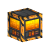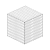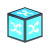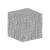# Randomizer

RandomizerHit Points15
Armor0.0%
Mass0.01
Luminositynone
Data Value (ID)979

Randomizers are logic blocks which have a 50% chance to change state when given either a high or a low logic signal. They can be used in series or parallel to create more complex probabilities.

## Item Description

"This block produces random results when activated. Each time it receives any signal (true or false) it changes it’s state by a 50:50 chance."

## Production

Production Info
Produced in a Standard FactoryRequiresTo create
Alloyed Metal Mesh
x2Randomizer
x1Crystal Composite
x2## Usage

Randomizers function in a similar manner to Flip Flops, in that when on, they remain on until caused to change state again. The key difference, of course, is that a Randomizer will not always change state when given an input. The chance, per input, of a Randomizer changing state is 50%. It is relevant to note that, for a Randomizer, an "input" actually constitutes any change in state of a block which is linked to it, not just high signals. This means that a Button produces two inputs to a Randomizer; one when the Button is activated, one when it turns off again.

While a single Randomizer has a constant 50% chance of changing state, more complex probabilities can be created by linking many Randomizers to a single logic gate.

Connecting several Randomizers (all with the same input) to a single AND-Signal should result in an output probability (from the AND-Signal) of (P = 0.5^n). For the AND-Signal to activate, of course, all of the Randomizers must turn on simultaneously; this behavior can be reasonably compared to multiplying along the branches of a probability tree to find the probability of a particular outcome.

Conversely, linking many Randomizers to an OR-Signal will result in an increased chance of activation. Since the OR-Signal will turn on when any of the Randomizers activate, the probability of an output is much higher than the same circuit using an AND-Signal. This configuration will result in an output probability (from the OR-Signal) of (P = 1 - 0.5^n) and will approach 1 as the number of randomizers increase. (Disclaimer: the exact behaviors of Randomizers are not yet fully tested, and this analogy may prove incorrect)

Increasingly complex probabilities can be created by combining various layers of such circuits, in varying proportions.